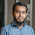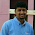# Sum of ODD Numbers in the Given Range

## Source Code

#include <stdio.h>

void main()
{
int index, begno, endno, sum = 0;
printf("Program for sum of odd numbers in the given range");

printf("Enter Beg. No.: ");
scanf("%d", &begno);
printf("Enter End. No.: ");
scanf("%d", &endno);
index = begno;
if( (begno % 2) == 0) // If it even, then make it ODD
index = begno + 1;
for(; index <= endno; index += 2)
{
sum = sum + index;
}
printf("The sum of odd numbers between %d and %d is: %d", begno, endno, sum);
}

## Output

Program for sum of odd numbers in the given range
Enter Beg. No.: 1
Enter End. No.: 100
The sum of odd numbers between 1 and 100 is: 2500

1.#include
main()
{
int i,n,sum=0;
printf("Enter the number for sum\n");
scanf("%d",&n);
for(i=1;i<=n;i++)
{
if(i%2!=0)
sum+=i;
}
printf("The sum of all odd number:%d\n",sum);
return 0;
}

2.#include"stdio.h"
main(){
int i,s,n,sum=0;
printf("Enter start num :\n");
scanf("%d",&s);
printf("\nEnter end num :\n");
scanf("%d",&n);
for(i=s;i<=n;i++){
if(i%2!=0){
sum+=i;
}
}
printf("Sum of odd num from given range is :%d",sum);
}

3.Get the simplest way of approach to this program with proper explanation at PROGRAMMING HUB

4.#include
main()
{
int a,b,i,sum=0;
printf("The start value : ");
scanf("%d",&a);
printf("\nThe end value : ");
scanf("%d",&b);
do
{

sum+=a;
a++;
}
while(a<=b);
printf("\nSum of all numbers in given range : %d\n",sum);
}